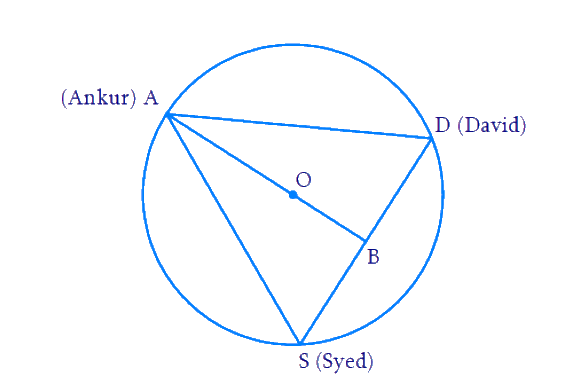# Ex.10.4 Q6 Circles Solution - NCERT Maths Class 9

Go back to  'Ex.10.4'

## Question

A circular park of radius $$20\,\rm m$$ is situated in a colony. Three boys Ankur, Syed and David are sitting at equal distance on its boundary each having a toy telephone in his hands to talk to each other. Find the length of string of each phone.

Video Solution
Circles
Ex 10.4 | Question 6

## Text Solution

What is known?

Three boys are on boundary of a circular park. Distance between them is equal. Radius of circular park is given.

What is unknown?

Length of string of each phone.

Reasoning:

Centre and Centroid are the same for an equilateral triangle. And it divides the median in the ratio \begin{align}2:1.\end{align} The median is also the perpendicular bisector for the opposite side.

Steps:Let \begin{align}{A, D, S}\end{align} denote the positions of Ankur, David and Syed respectively.

\begin{align}\Delta{ADS}\end{align} is an equilateral triangle since all the $$3$$ boys are on equidistant from one another.

Let $${B}$$ denote the mid-point of $${DS}$$ and hence $${AB}$$ is the median and perpendicular bisector of $${DS.}$$ Hence $$\Delta {.ABS}$$ is a right angled triangle with \begin {align} \angle {ABS}=90^{\circ} \end {align}

$$O$$ (centroid) divides the line $${AB}$$ in the ratio $$\text{2:1.}$$ So $${OA : OB = 2 : 1.}$$

\begin{align}\frac{{OA}}{{OB}} &= \frac{2}{1}\,\end{align}

\begin{align} \text{Since } OA &= 20 \; \rm {then}\\OB &=10\rm{m}\end{align}

\begin {align} {AB}&={OA}+{OB}\\ &=20+10 \\&=30 \, \rm{m}\qquad \ldots .(1) \end {align}

Let the side of equilateral triangle $$\Delta {ADS}$$ be $$2x.$$

\begin{align}{AD}&={DS} \\ &={SA}\\ &=2 x \ldots .(2) \end {align}

Since $${B}$$ is the mid-point of $${DS,}$$ we get

\begin {align}{BS}={BD}=x \dots(3)\end {align}

Applying Pythagoras theorem to $$\Delta {ABS,}$$ we get:

\begin {align}{AD}^{2}&={AB}^{2}+{BD}^{2} \\ {(2 x)^{2}}&={30^{2}+x^{2}} \\ 4 x^{2}&=900+x^{2}\\ 3 x^{2}&=900 \\ x^{2}&=\frac{900}{3}=300 \\ x&=\sqrt{300} \\ &=17.32 \, \rm {m}\end{align}

\begin {align}{AD}={DS}={SA}=2 x=34.64 \, \mathrm{m} \end {align}

Length of the string $$=$$ Distance between them

$$= AD$$ or $$DS$$ or $$SA = 34.64\,\rm m.$$

Video Solution
Circles
Ex 10.4 | Question 6

Learn from the best math teachers and top your exams

• Live one on one classroom and doubt clearing
• Practice worksheets in and after class for conceptual clarity
• Personalized curriculum to keep up with school